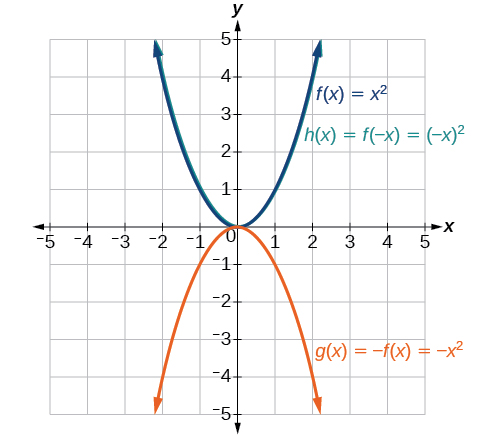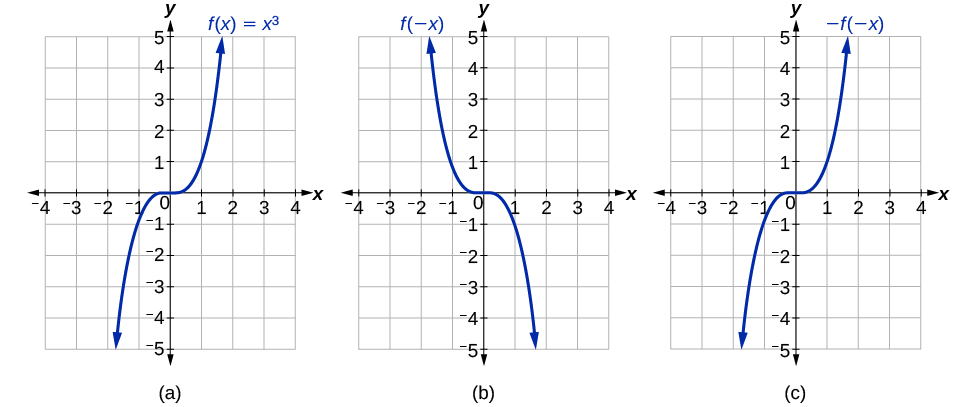# 3.5 Transformation of functions  (Page 6/21)

 Page 6 / 21

Given the toolkit function $\text{\hspace{0.17em}}f\left(x\right)={x}^{2},\text{\hspace{0.17em}}$ graph $\text{\hspace{0.17em}}g\left(x\right)=-f\left(x\right)\text{\hspace{0.17em}}$ and $\text{\hspace{0.17em}}h\left(x\right)=f\left(-x\right).\text{\hspace{0.17em}}$ Take note of any surprising behavior for these functions.Notice: $\text{\hspace{0.17em}}g\left(x\right)=f\left(-x\right)\text{\hspace{0.17em}}$ looks the same as $\text{\hspace{0.17em}}f\left(x\right)$ .

## Determining even and odd functions

Some functions exhibit symmetry so that reflections result in the original graph. For example, horizontally reflecting the toolkit functions $f\left(x\right)={x}^{2}$ or $f\left(x\right)=|x|$ will result in the original graph. We say that these types of graphs are symmetric about the y -axis. A function whose graph is symmetric about the y -axis is called an even function.

If the graphs of $\text{\hspace{0.17em}}f\left(x\right)={x}^{3}\text{\hspace{0.17em}}$ or $\text{\hspace{0.17em}}f\left(x\right)=\frac{1}{x}\text{\hspace{0.17em}}$ were reflected over both axes, the result would be the original graph, as shown in [link] .(a) The cubic toolkit function (b) Horizontal reflection of the cubic toolkit function (c) Horizontal and vertical reflections reproduce the original cubic function.

We say that these graphs are symmetric about the origin. A function with a graph that is symmetric about the origin is called an odd function .

Note: A function can be neither even nor odd if it does not exhibit either symmetry. For example, $\text{\hspace{0.17em}}f\left(x\right)={2}^{x}\text{\hspace{0.17em}}$ is neither even nor odd. Also, the only function that is both even and odd is the constant function $\text{\hspace{0.17em}}f\left(x\right)=0.$

## Even and odd functions

A function is called an even function    if for every input $\text{\hspace{0.17em}}x$

$f\left(x\right)=f\left(-x\right)$

The graph of an even function is symmetric about the $y\text{-}$ axis.

A function is called an odd function    if for every input $\text{\hspace{0.17em}}x$

$f\left(x\right)=-f\left(-x\right)$

The graph of an odd function is symmetric about the origin.

Given the formula for a function, determine if the function is even, odd, or neither.

1. Determine whether the function satisfies $\text{\hspace{0.17em}}f\left(x\right)=f\left(-x\right).\text{\hspace{0.17em}}$ If it does, it is even.
2. Determine whether the function satisfies $\text{\hspace{0.17em}}f\left(x\right)=-f\left(-x\right).\text{\hspace{0.17em}}$ If it does, it is odd.
3. If the function does not satisfy either rule, it is neither even nor odd.

## Determining whether a function is even, odd, or neither

Is the function $\text{\hspace{0.17em}}f\left(x\right)={x}^{3}+2x\text{\hspace{0.17em}}$ even, odd, or neither?

Without looking at a graph, we can determine whether the function is even or odd by finding formulas for the reflections and determining if they return us to the original function. Let’s begin with the rule for even functions.

$f\left(-x\right)={\left(-x\right)}^{3}+2\left(-x\right)=-{x}^{3}-2x$

This does not return us to the original function, so this function is not even. We can now test the rule for odd functions.

$-f\left(-x\right)=-\left(-{x}^{3}-2x\right)={x}^{3}+2x$

Because $\text{\hspace{0.17em}}-f\left(-x\right)=f\left(x\right),\text{\hspace{0.17em}}$ this is an odd function.

Is the function $\text{\hspace{0.17em}}f\left(s\right)={s}^{4}+3{s}^{2}+7\text{\hspace{0.17em}}$ even, odd, or neither?

even

## Graphing functions using stretches and compressions

Adding a constant to the inputs or outputs of a function changed the position of a graph with respect to the axes, but it did not affect the shape of a graph. We now explore the effects of multiplying the inputs or outputs by some quantity.

We can transform the inside (input values) of a function or we can transform the outside (output values) of a function. Each change has a specific effect that can be seen graphically.

## Vertical stretches and compressions

When we multiply a function by a positive constant, we get a function whose graph is stretched or compressed vertically in relation to the graph of the original function. If the constant is greater than 1, we get a vertical stretch ; if the constant is between 0 and 1, we get a vertical compression . [link] shows a function multiplied by constant factors 2 and 0.5 and the resulting vertical stretch and compression.

#### Questions & Answers

A laser rangefinder is locked on a comet approaching Earth. The distance g(x), in kilometers, of the comet after x days, for x in the interval 0 to 30 days, is given by g(x)=250,000csc(π30x). Graph g(x) on the interval [0, 35]. Evaluate g(5)  and interpret the information. What is the minimum distance between the comet and Earth? When does this occur? To which constant in the equation does this correspond? Find and discuss the meaning of any vertical asymptotes.
Kaitlyn Reply
The sequence is {1,-1,1-1.....} has
amit Reply
circular region of radious
Kainat Reply
how can we solve this problem
Joel Reply
Sin(A+B) = sinBcosA+cosBsinA
Eseka Reply
Prove it
Eseka
Please prove it
Eseka
hi
Joel
June needs 45 gallons of punch. 2 different coolers. Bigger cooler is 5 times as large as smaller cooler. How many gallons in each cooler?
Arleathia Reply
7.5 and 37.5
Nando
find the sum of 28th term of the AP 3+10+17+---------
Prince Reply
I think you should say "28 terms" instead of "28th term"
Vedant
the 28th term is 175
Nando
192
Kenneth
if sequence sn is a such that sn>0 for all n and lim sn=0than prove that lim (s1 s2............ sn) ke hole power n =n
SANDESH Reply
write down the polynomial function with root 1/3,2,-3 with solution
Gift Reply
if A and B are subspaces of V prove that (A+B)/B=A/(A-B)
Pream Reply
write down the value of each of the following in surd form a)cos(-65°) b)sin(-180°)c)tan(225°)d)tan(135°)
Oroke Reply
Prove that (sinA/1-cosA - 1-cosA/sinA) (cosA/1-sinA - 1-sinA/cosA) = 4
kiruba Reply
what is the answer to dividing negative index
Morosi Reply
In a triangle ABC prove that. (b+c)cosA+(c+a)cosB+(a+b)cisC=a+b+c.
Shivam Reply
give me the waec 2019 questions
Aaron Reply

### Read also:

#### Get the best Algebra and trigonometry course in your pocket!

Source:  OpenStax, Algebra and trigonometry. OpenStax CNX. Nov 14, 2016 Download for free at https://legacy.cnx.org/content/col11758/1.6
Google Play and the Google Play logo are trademarks of Google Inc.

Notification Switch

Would you like to follow the 'Algebra and trigonometry' conversation and receive update notifications?ByByByByBy